Request a Tool

Weber Number Calculator

The Weber Number is especially used for multiphase flows with strongly curved surfaces.

Density
0

Formula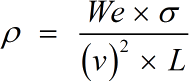• ρ = Density
• v = Velocity
• L = Characteristic Length
• σ = Surface Tension
• We = Weber Number

Defination / Uses

Density is the mass of a material substance per unit volume. Density is calculated using the formula d = M/V, where d represents density, M is mass, and V is volume. The density of a substance is usually measured in grammes per cm³.

The Weber number is termed as a dimensionless number in fluid mechanics which is often used in analysing fluid flows where there is an interface between two different fluids.

Velocity
0

Formula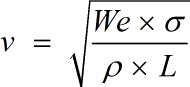• v = Velocity
• L = Characteristic Length
• σ = Surface Tension
• We = Weber Number
• ρ = Density

Defination / Uses

The concept of velocity for an object is the rate at which the object's position changes in relation to a frame of reference and time. It's a vector quantity, which means we need both magnitude (speed) and direction (orientation) to define velocity. Its SI unit is meter per second (m/s). When the amount or direction of a body's velocity changes, the body is said to be accelerating.

The Weber number is termed as a dimensionless number in fluid mechanics which is often used in analysing fluid flows where there is an interface between two different fluids.

Output

Characteristic Length
0

Formula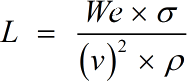• L = Characteristic Length
• σ = Surface Tension
• We = Weber Number
• ρ = Density
• v = Velocity

Defination / Uses

Characteristic length is a crucial dimension in physics that specifies the scale of a physical system. Characteristic length is defined in computational mechanics to force localization of a stress softening constitutive equation. An integration point is referred to the length.

The Weber number is termed as a dimensionless number in fluid mechanics which is often used in analysing fluid flows where there is an interface between two different fluids.

Surface Tension
0

Formula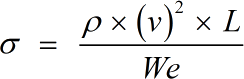• σ = Surface Tension
• We = Weber Number
• ρ = Density
• v = Velocity
• L = Characteristic Length

Defination / Uses

The ability of fluid surfaces to shrink to the smallest feasible surface area is known as surface tension. Have you ever noticed that even after you've filled a glass halfway with water, you can still add a few more drops till it overflows? Have you ever broken a thermometer and watched how the mercury reacts when it falls? All of this occurs as a result of the surface's surface tension.

The Weber number is termed as a dimensionless number in fluid mechanics which is often used in analysing fluid flows where there is an interface between two different fluids.

Weber Number
0

Formula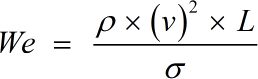• We = Weber Number
• ρ = Density
• v = Velocity
• L = Characteristic Length
• σ = Surface Tension

Defination / Uses

In fluid mechanics, the Weber number is a characteristic number. It depicts the ratio between deforming inertial forces and stabilising cohesive forces for liquids moving across a fluid medium as a dimensionless number.

Spray droplets disperse more easily and oil drops in an aqueous environment split apart more easily as the deforming force increases due to a greater speed or longer process length. This process is slowed down by a high surface or interfacial tension.

Spray droplets disperse more easily and oil drops in an aqueous environment split apart more easily as the deforming force increases due to a greater speed or longer process length. This process is slowed down by a high surface or interfacial tension.